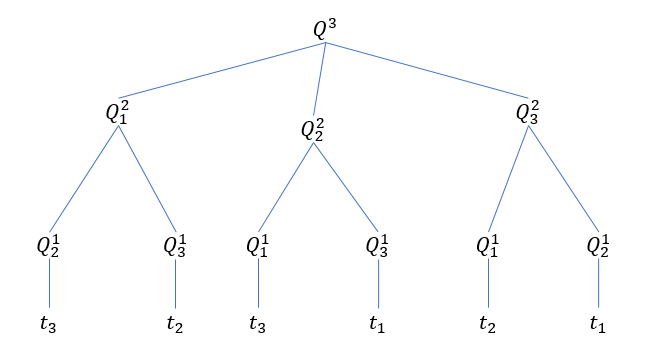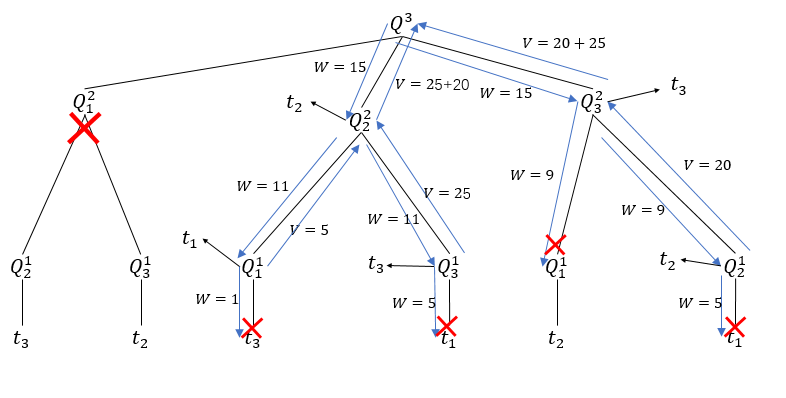Fenrier Lab

# 算法题：背包问题

### 质量约束

$V^n = f(W, R^n)$

$Q_1^{n-1},Q_2^{n-1},Q_3^{n-1},,,Q_n^{n-1}$

$V_j^{n-1} = f(W-w_j, R^n\setminus t_j)$

1、$$S^n \setminus t_j$$ 的总价值比 $$S_j^{n-1}$$ 的更小，此时 $$S_j^{n-1} \cup t_j$$ 将是问题 $$Q^n$$ 更好的解。

2、$$S^n \setminus t_j$$ 的总重量比 $$W-w_j$$ 更大，此时 $$S^n$$ 的总重量将超过 $$W$$。

$f(W, R^n) =\max_j\,\, \left(f(W-w_j, R^n\setminus t_j) + v_j\right)$func remove_problem_list(items)
remove_list = [];
sort(items);
n = items.length;
for i: n -> 1
base_weight = items[i].weight;
for j: i -> 1
if items[j].weight < base_weight
remove_list.append(items[i]);
break;
end
end
end
return remove_list;
end


$t_1=(10, 5)\\t_2=(4,20)\\t_3=(6,25)$func solve(W, items)
## 处理平凡情况
if items.length == 1
if items.weight <= W
return items.value;
else
return 0;
end
end

remove_problem_list = remove_problem_list(items);
select_item_list = item not in remove_problem_list;

values = [];
for item in select_item_list
values.append(solve(W - item.weight, items except item) + item.value);
end

return max in values;

end


func solve(W, items)
## 处理平凡情况
if items.length == 1
if items.weight <= W
return items;
else
return [];
end
end

remove_problem_list = remove_problem_list(items);
select_item_list = items not in remove_problem_list;

candicate_items_list = [];
for item in select_item_list
candicate_items_list.append(solve(W - item.weight, items except item).append(item));
end

return candicate_items in candicate_items_list which have max value

end


//定义物品类 Item
class Item{
int w;
int v;
Item(int w, int v) {
this.w = w;
this.v = v;
}
public String toString(){
return w+":" +v;
}
}

//移除不必要问题的方法
private List<Item> removeProblemList(List<Item> items, int W) {
ArrayList<Item> remove = new ArrayList<>();
for(int i = items.size() - 1; i >= 0;  i--) {
Item base = items.get(i);
if(base.w > W) {
}else{
for(int j = i - 1; j >= 0; j--) {
if(base.w > items.get(j).w) {
break;
}
}
}
}
return remove;
}

//递归调用方法
public List<Item> solve(int W, List<Item> items) {
if(W <= 0) {
return new ArrayList<>();
}
if(items.size() == 1) {
if(items.get(0).w <= W) {
ArrayList<Item> candicate = new ArrayList<>();
return candicate;
}else{
return new ArrayList<>();
}
}

List<Item> remove = removeProblemList(items, W);
//待求解问题
List<Item> selects = items.stream()
.filter(item -> !remove.contains(item))
.collect(Collectors.toList());

ArrayList<List<Item>> candicate_items_list = new ArrayList<>();

for (Item select : selects) {
List<Item> remain = items.stream().filter(item -> item != select).collect(Collectors.toList());
List<Item> result = solve(W - select.w, remain);
}

//从候选列表中找到具有最大价值的物品路径
int max = 0;
int max_index=0;
for (int i = 0; i < candicate_items_list.size(); i++) {
int value = candicate_items_list.get(i).stream().mapToInt(item -> item.v).sum();
if(value > max){
max = value;
max_index = i;
}
}
return candicate_items_list.get(max_index);
}


@Test
public void testKP() {
Item[] items = {new Item(3,4),new Item(3,4),new Item(3,4),
new Item(8, 5),new Item(8, 5),new Item(2, 2),new Item(2, 2),
new Item(5, 3),new Item(20, 11)};
sort(items);
int W = 60;
List<Item> solution = solve(W, Arrays.asList(items));
System.out.println(solution);
//output: [2:2, 2:2, 5:3, 3:4, 3:4, 3:4, 8:5, 8:5, 20:11]
}